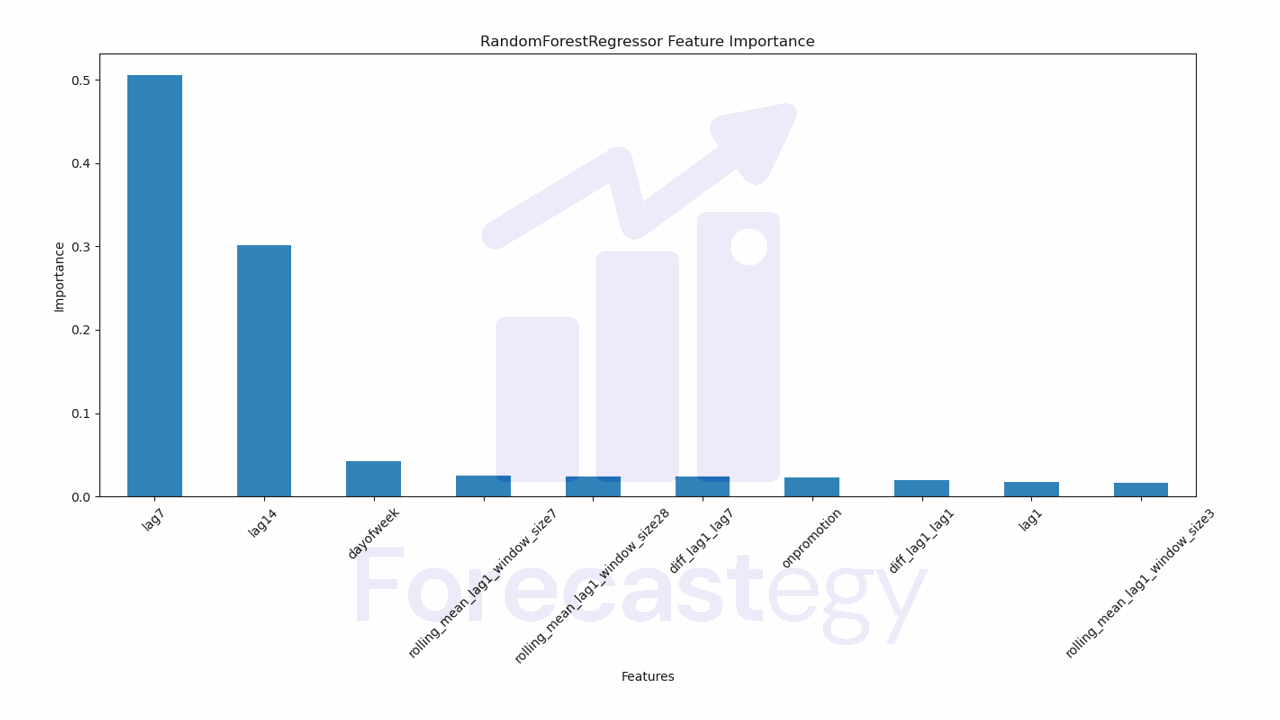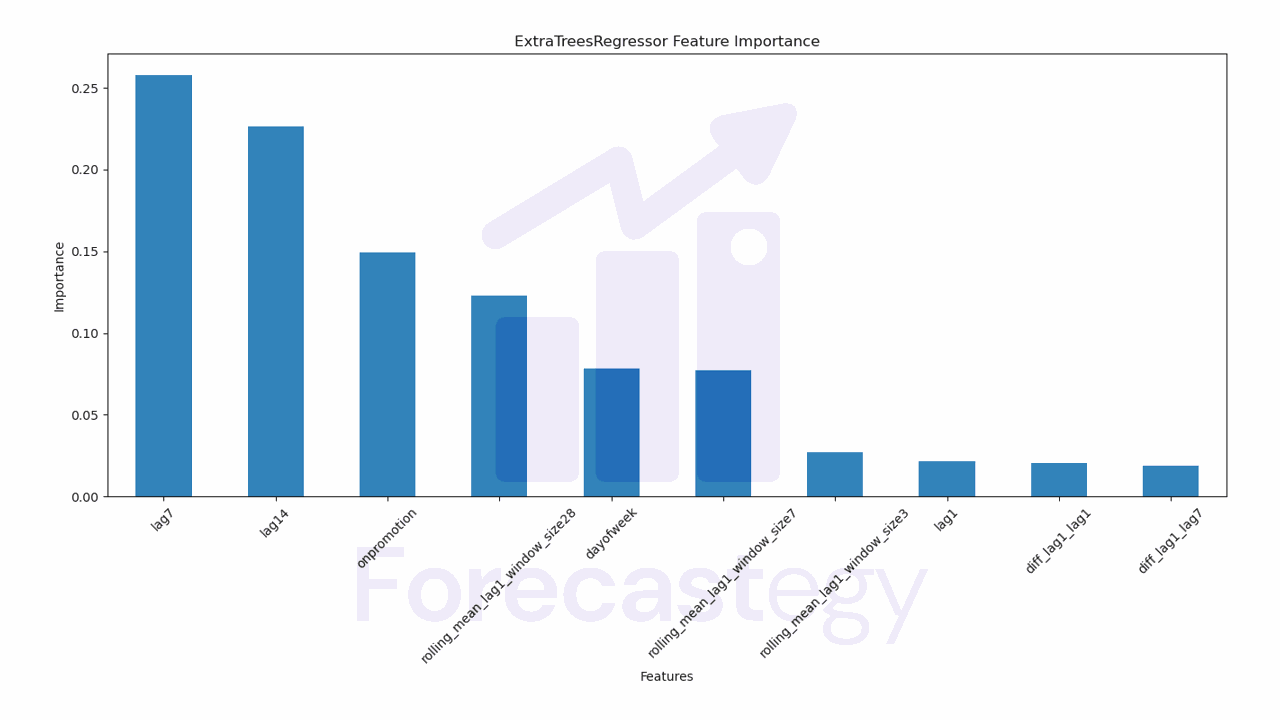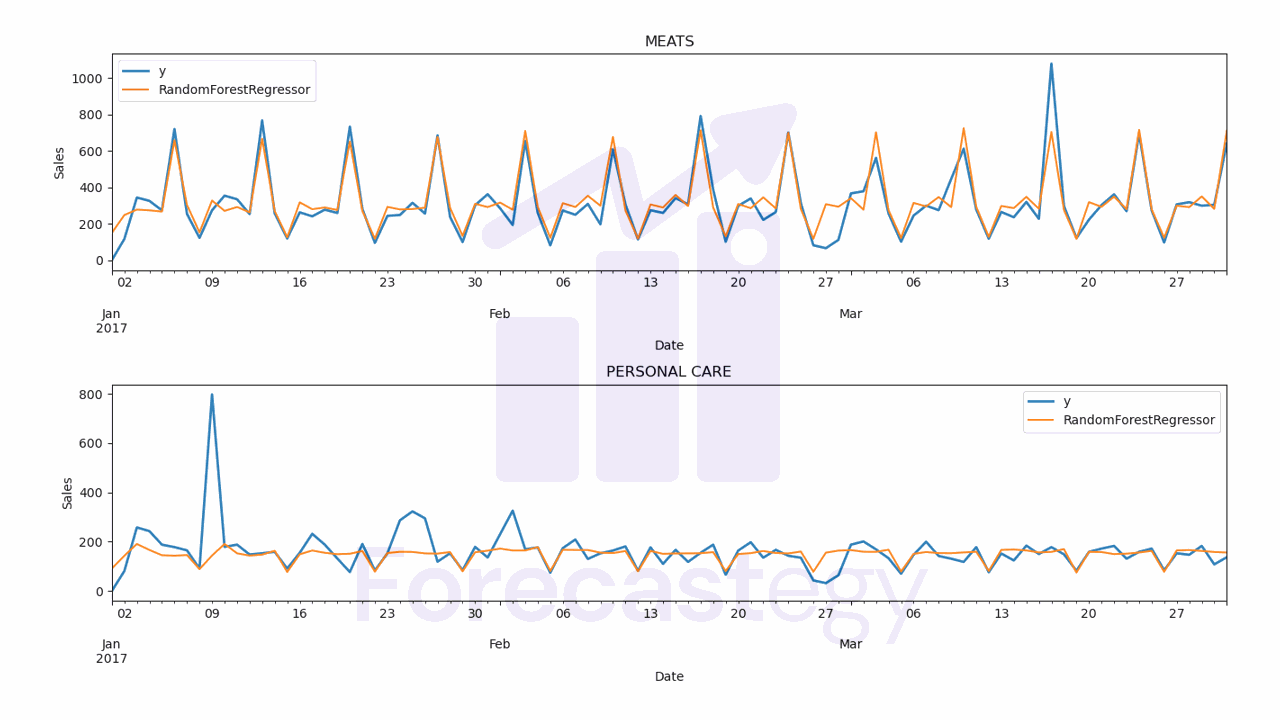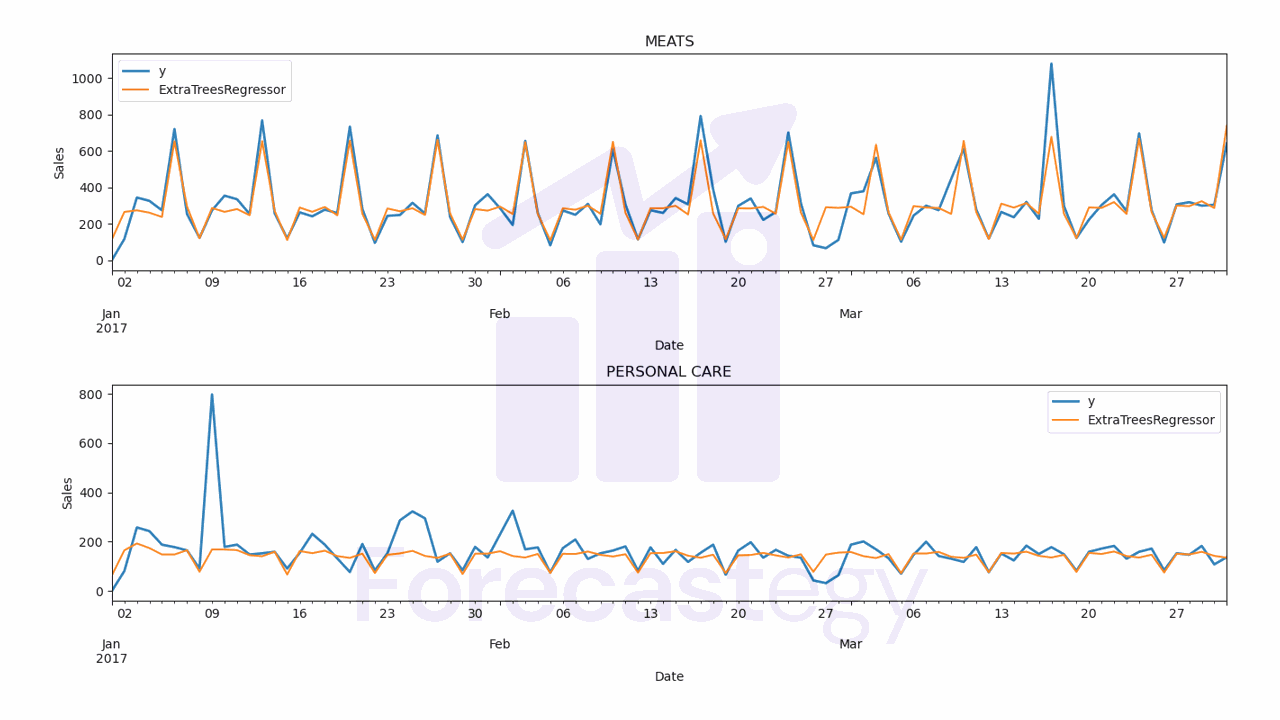Forecasting time series is a very common task in the daily life of a data scientist, which is surprisingly little covered in beginner machine learning courses.

It can be predicting future demand for a product, city traffic or even the weather.

With accurate time series forecasts, companies can adjust their production strategies, inventory management, resource allocation and other key decisions, leading to significant cost reduction and increased efficiency.

Furthermore, forecasts also allow companies to be more proactive rather than reactive, anticipating market trends and adjusting their strategies accordingly.

In this article you will learn how to forecast multiple time series easily with scikit-learn and the mlforecast library!

## Installing MLForecast and Scikit-Learn#

Instead of wasting time and making mistakes in manual data preparation, let’s use the mlforecast library.

It has tools that transform our raw time series data into the correct format for training and prediction with scikit-learn.

It computes the main features we want when modeling time series, such as aggregations over sliding windows, lags, differences, etc.

Finally, it implements a recursive prediction loop to forecast multiple steps into the future.

I will explain more about all this in the course of the article.

You can install the library with pip:

``````pip install mlforecast
pip install matplotlib
``````

It will install scikit-learn, numpy and pandas too.

We will also need `matplotlib` to visualize the results.

The library is available in the Anaconda repository, but I recommend installing the pip version as it has the latest version.

## How To Prepare Time Series Data for Scikit-Learn#

We will use real sales data made available by Favorita, a large Ecuadorian grocery chain.

We have sales data from 2013 to 2017 for multiple stores and product categories.

``````import pandas as pd
import numpy as np
from matplotlib import pyplot as plt

path = 'train.csv'
``````

To run this example faster, we will only use the data from one store and two product categories.

So we have 2 time series to forecast, but this code can be used with as many time series as you want.

``````data2 = data.loc[(data['store_nbr'] == 1) & (data['family'].isin(['MEATS', 'PERSONAL CARE'])), ['date', 'family', 'sales', 'onpromotion']]
``````

This data doesn’t contain a record for December 25, so I just copied the sales from December 18 to December 25 to keep the weekly pattern.

``````dec25 = list()
for year in range(2013,2017):
for family in ['MEATS', 'PERSONAL CARE']:
dec18 = data2.loc[(data2['date'] == f'{year}-12-18') & (data2['family'] == family)]
dec25 += [{'date': pd.Timestamp(f'{year}-12-25'), 'family': family, 'sales': dec18['sales'].values, 'onpromotion': dec18['onpromotion'].values}]
data2 = pd.concat([data2, pd.DataFrame(dec25)], ignore_index=True).sort_values('date')

data2 = data2.rename(columns={'date': 'ds', 'sales': 'y', 'family': 'unique_id'})
``````

The columns are:

• `date`: date of the record
• `family`: product category
• `sales`: number of sales
• `onpromotion`: number of products of that category that were on promotion on that day

To keep Nixtla’s (the creator of `mlforecast`) libraries standard format, let’s rename the columns to `ds` (date), `y` (target) and `unique_id` (family).

`unique_id` should identify each time series you have.

If we had more than one store, we would have to add the store number along with the categories to `unique_id`.

An example would be `unique_id = store_nbr + '_' + family`.

This is the final version of our dataframe `data2`:

ds unique_id y onpromotion
2013-01-01 00:00:00 MEATS 0 0
2013-01-01 00:00:00 PERSONAL CARE 0 0
2013-01-02 00:00:00 MEATS 369.101 0
2013-01-02 00:00:00 PERSONAL CARE 194 0
2013-01-03 00:00:00 MEATS 272.319 0

A row for each record containing the date, the time series ID (`family` in our example), the target value and columns for external variables (`onpromotion`).

Notice the time series records are stacked on top of each other.

Let’s split the data into train and validation sets.

## Time Series Validation#

You should never use random or k-fold validation for time series.

That would cause data leakage, as you would be using future data to train your model.

In practice, you can’t take random samples from the future to train your model, so you can’t use them here.

To avoid this issue, we will use a simple time series split between past and future.

A career tip: knowing how to do time series validation correctly is a skill that will set you apart from many data scientists (even experienced ones!).

Our training set will be all the data between 2013 and 2016 and our validation set will be the first 3 months of 2017.

``````train = data2.loc[data2['ds'] < '2017-01-01']
valid = data2.loc[(data2['ds'] >= '2017-01-01') & (data2['ds'] < '2017-04-01')]
``````

## Feature Engineering for Time Series Forecasting#

Now that we have our data formatted according to what `mlforecast` expects, let’s define the features we are going to use.

This is a crucial step for the success of your model.

I usually think of 4 main types of features for time series. You can calculate them using the target or external variables:

### Lags#

Lags are simply past values of the time series that you shift forward to use as features in the model.

For example, if you want to predict the demand of a product next week, you can use the demand of the same weekday in the previous week as a feature.

In practice, the demand of a product on a given day of the week is similar in different weeks, so this feature can be useful for the model.

You can also use longer lags, like the demand of the same day in the previous month or year.

It’s important to test different lags to find the ones that work best for your specific problem.

Don’t be afraid of adding lots of lag features!

No one can tell you which lags are the best for your problem without testing.

### Rolling Window Aggregations#

Rolling window aggregations are statistical functions applied to records in a sliding window.

They can be calculated over expanding windows too, but sliding windows are usually more robust in practice.

An example is calculating the average daily demand of the last 7 days, or the standard deviation of the returns of a financial asset over the last 4 weeks.

These features can help the model identify trends in the time series.

Like lags, it’s important to test different aggregation functions and window sizes to find the ones that work best for your specific problem.

### Date Components#

Date components are values you extract from a specific date or timestamp.

For example, you can include the hour of the day, the day of the week, the month, the season of the year, etc.

These features can help the model identify seasonal and cyclical patterns in the time series.

As with other features, test different components and find the ones that work best.

### Differences#

Differences are simply the subtraction of two values in a time series.

An example is the difference between the demand of today and the demand of yesterday.

Even though we have lags, having differences as features can bring specific information the model needs to more easily identify patterns.

Feature engineering is a lot about making the patterns in the data more explicit for the model.

### Feature Engineering Code#

Now that we know the features we want to use, let’s compute them with the `mlforecast` library.

First, we need to create a list with the scikit-learn models we want to test.

``````from sklearn.ensemble import RandomForestRegressor, ExtraTreesRegressor

models = [RandomForestRegressor(random_state=0, n_estimators=100),
ExtraTreesRegressor(random_state=0, n_estimators=100)]
``````

I will test only 2 models, the Random Forest and the Extra Trees, but you can test as many models as you want. Just add them to the list.

At the time of writing, `mlforecast` and `window_ops` don’t support calculating difference features, so I created the `diff` function using `numba`.

``````from numba import njit

@njit
def diff(x, lag):
x2 = np.full_like(x, np.nan)
for i in range(lag, len(x)):
x2[i] = x[i] - x[i-lag]
return x2
``````

`numba` is a library that optimizes your Python code to run faster and it’s recommended by the `mlforecast` developers when creating custom functions to compute features.

We need to add the `@njit` decorator to the function to tell `numba` to compile it.

The function gets two arguments, `x` and `lag`.

`x` is an array and `lag` is the number of periods we want to use to calculate the difference.

The first line inside the function creates a new array `x2` with the same format as the array `x`, but all the values are initialized as NaN.

Inside the loop, we will set the values of `x2` to the difference between the values in `x` and the values in `x` shifted by `lag` periods.

If it’s a lag of 3, for example, the value in position 3 of `x2` is the difference between the value in position 3 and the value in position 0 of `x`.

The function returns the array `x2` with the differences for each period.

Now we will create the object that manages the creation of the features and the training of the models.

``````from window_ops import rolling_mean
from mlforecast import MLForecast

model = MLForecast(models=models,
freq='D',
lags=[1,7,14],
lag_transforms={
1: [(rolling_mean, 3), (rolling_mean, 7), (rolling_mean, 28), (diff, 1), (diff, 7)],
},
date_features=['dayofweek'],
``````

`MLForecast` is the object that will make our work easier.

It manages feature engineering and model training.

The first argument, `models`, is the list with the models we want to train.

The second is `freq`, which indicates the frequency of the time series. Daily in our example.

The third argument, `lags=[1,7,14]`, indicates the lag values we want to use as features. We will use lags of 1, 7 and 14 days.

The fourth argument, `lag_transforms={...}`, is a dictionary with the functions we want to apply to the lags.

The keys in the dictionary are the lag series to which the functions will be applied.

We will calculate a rolling mean of lag 1 with windows of 3, 7 and 28 days, and the difference between the current value and the value 1 and 7 days before.

Just remember that “current value” is the series value at the lag, not the value of the current time step, as the latter is the value we want to predict.

In our example, the 7-day rolling mean is computed using lag 1 as the most recent value, and so on.

The functions will get an array with the original time series shifted by the lag value in the same order as the original dataframe.

Usually it’s from the oldest record to the newest.

Then we set `date_features=['dayofweek']` to specify which date components we want to extract. I used only the day of the week.

Finally, we set `num_threads=6` to specify how many threads should be used to process the data in parallel.

I used 6 because I have a 6-core CPU, but you can use as many threads as you want.

Remember that we still have the `onpromotion` feature, which is an external variable related to the target.

Any columns besides the target, the ID and the date will be considered as external variables.

By default it considers them as static features, which means they don’t change over time (like a product brand or the store city).

During prediction it generates a dataframe with the same values for all the time steps.

We can explicitly tell which columns are static features with the `static_features` argument in the `fit` method.

Even if you don’t have any static features but have dynamic features, it’s important to pass an empty list to this argument, because `MLForecast` will think your dynamic features are static and ignore its new values if you don’t do that.

``````model.fit(train, id_col='unique_id', time_col='ds', target_col='y', static_features=[])

p = model.predict(horizon=90, dynamic_dfs=[valid[['unique_id', 'ds', 'onpromotion']]])
p = p.merge(valid[['unique_id', 'ds', 'y']], on=['unique_id', 'ds'], how='left')
``````

In the `fit` method, we pass the training dataframe, the names of the columns with the ID of the time series, date, target, and the list with the names of static features.

In the `predict` method, we pass the number of time steps we want to predict (horizon) and a list with dataframes with the values of the dynamic features for the periods we want to predict.

The number of time steps is counted from the last date in the training dataframe.

In our case, we know which products will be on promotion in the next 90 days (we can plan it), so we can pass a dataframe with the `onpromotion` column for the next 90 days.

We could even use this model to simulate the impact of a promotion on sales by setting the `onpromotion` column to `1` for arbitrary periods in the future.

But if we had external variables that we don’t know in advance (like temperature), we would need to use estimates.

A very common mistake is to use historical values of the external variables during validation without considering that they won’t be available at the time of prediction in production.

This shows you an overly optimistic estimate of the model performance, which won’t be real in practice.

That’s why it’s important to create these features here using the same process that will be used in production.

It’s important that the dataframes with the external variables have columns that can be used to merge it with the main dataframe.

At a minimum the date of the record and potentially any others that ID the time series.

Thinking about temperature again, we could have the city code as a static feature, and an external variables dataframe with the city code, date and temperature estimates for the prediction period.

The last line merges the predictions with the validation dataframe to make it easier to calculate the error and visualize the results.

The predictions for each model are stored in a column with the name of the model object.

unique_id ds RandomForestRegressor ExtraTreesRegressor y
MEATS 2017-01-01 00:00:00 149.719 115.594 0
PERSONAL CARE 2017-01-01 00:00:00 83.1048 64.9456 0
PERSONAL CARE 2017-01-02 00:00:00 138.117 154.29 81
MEATS 2017-01-02 00:00:00 245.477 267.907 116.724
MEATS 2017-01-03 00:00:00 274.266 267.524 344.583

## Checking For Data Leakage in Features#

It’s very important to check if the features were computed correctly, without data leakage (like using the target instead of a lag to compute a difference).

We can use the `preprocess` method. It returns a dataframe with the features computed for the training data.

``````model.preprocess(train, id_col='unique_id', time_col='ds', target_col='y', static_features=[])
``````
ds unique_id y onpromotion lag1 lag7 lag14 rolling_mean_lag1_window_size3 rolling_mean_lag1_window_size7 rolling_mean_lag1_window_size28 diff_lag1_lag1 diff_lag1_lag7 dayofweek
2013-01-29 00:00:00 MEATS 260.633 0 325.072 274.261 287.346 289.11 311.035 296.329 57.256 50.186 1
2013-01-29 00:00:00 PERSONAL CARE 91 0 107 90 95 88.6667 88.7143 98.5357 65 18 1
2013-01-30 00:00:00 MEATS 304.018 0 260.633 312.199 369.492 284.507 309.088 305.637 -64.439 -13.628 2
2013-01-30 00:00:00 PERSONAL CARE 98 0 91 103 118 80 88.8571 101.786 -16 1 2
2013-01-31 00:00:00 MEATS 264.937 0 304.018 215.607 280.879 296.574 307.92 303.313 43.385 -8.181 3

Pick a few samples and manually compute the values of the features in the original series to check if the values computed by `MLForecast` are correct.

## Recursive vs Direct Forecasting#

There are at least 3 different ways to generate forecasts when you use machine learning for time series.

The default way in `MLForecast` is to use the recursive or auto-regressive method.

To understand this method, imagine a time series with only 10 observations and a model trained to predict only 1 step ahead.

To get predictions for multiple periods, we will add the next step prediction into the original series as if it was a new sample and use the model to predict the next period.

We repeat these steps for the next periods until we have predictions for the full horizon we want to predict

As an alternative, we can use the direct method, which trains a separate, individual model, for each step of the forecast horizon.

So if we want to predict 10 periods, we train 10 models, each one to predict a specific step.

The recursive method is faster to train, especially if you have a large forecast horizon.

There is no way to know which method is better without testing, so if you need the best performance, even if the computational cost is high, test both.

To use the direct method in `MLForecast`, just pass the `max_horizon` argument in the `fit` method with the number of periods you want to predict.

``````model.fit(train, id_col='unique_id', time_col='ds', target_col='y',
static_features=[], max_horizon=90)
``````

## Retrieving Feature Importances#

To understand how the model is making its decisions, we can use the internal calculation of feature importances.

It’s very simple to get them using the `feature_importances_` attribute of the model.

``````pd.Series(model.models_['RandomForestRegressor'].feature_importances_, index=model.ts.features_order_).sort_values(ascending=False).plot.bar(
figsize=(1280/96,720/96), title='RandomForestRegressor Feature Importance', xlabel='Features', ylabel='Importance')
``````

Here we will see a bar chart with the features on the X axis and their importance on the Y axis. A higher bar means the model considers this feature more important when generating the forecast.## How To Measure Forecast Accuracy#

We can compute the error using any metric we want, as we do when normally using scikit-learn.

I created a custom function to calculate WMAPE, which is an adaptation of the mean absolute percentage error that solves the problem of dividing by zero.

The weight of each sample is given by the magnitude of the real value.

Here’s the function for the simplified version of the metric:

``````def wmape(y_true, y_pred):
return np.abs(y_true - y_pred).sum() / np.abs(y_true).sum()

print(f"WMAPE RandomForestRegressor: {wmape(p['y'], p['RandomForestRegressor'])}\nWMAPE ExtraTreesRegressor: {wmape(p['y'], p['ExtraTreesRegressor'])}")
``````

In our dataset, RandomForestRegressor had an error of 18.78% and ExtraTreesRegressor got 17.98%.The code to plot the forecast:

``````for model in ['RandomForestRegressor', 'ExtraTreesRegressor']:
fig,ax = plt.subplots(2,1, figsize=(1280/96, 720/96))
for ax_, family in enumerate(['MEATS', 'PERSONAL CARE']):
p.loc[p['unique_id'] == family].plot(x='ds', y='y', ax=ax[ax_], label='y', title=family, linewidth=2)
p.loc[p['unique_id'] == family].plot(x='ds', y=model, ax=ax[ax_], label=model)
ax[ax_].set_xlabel('Date')
ax[ax_].set_ylabel('Sales')
fig.tight_layout()
``````

The only change is that your `unique_id` column will be the SKU. You can use the rest of the code as is.